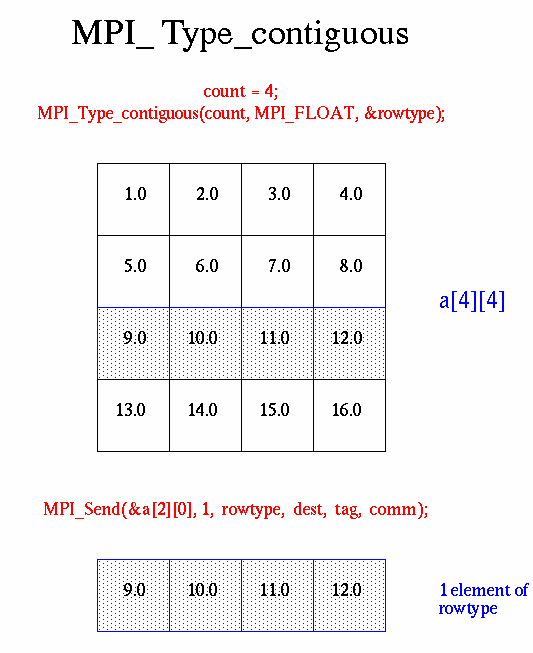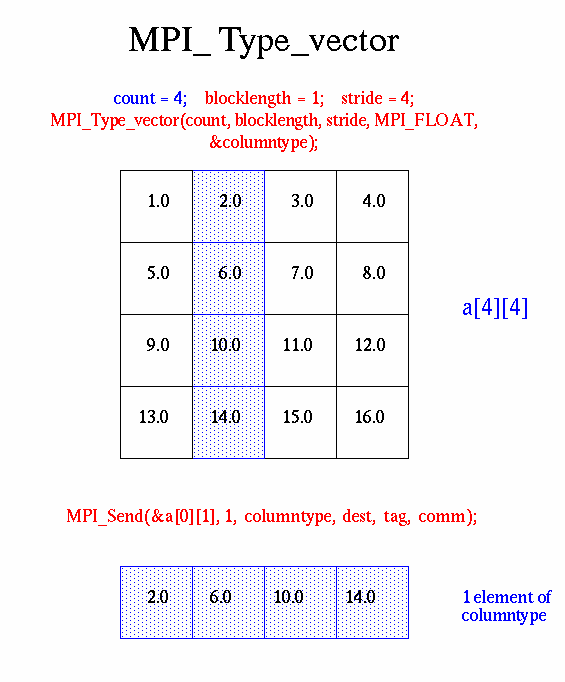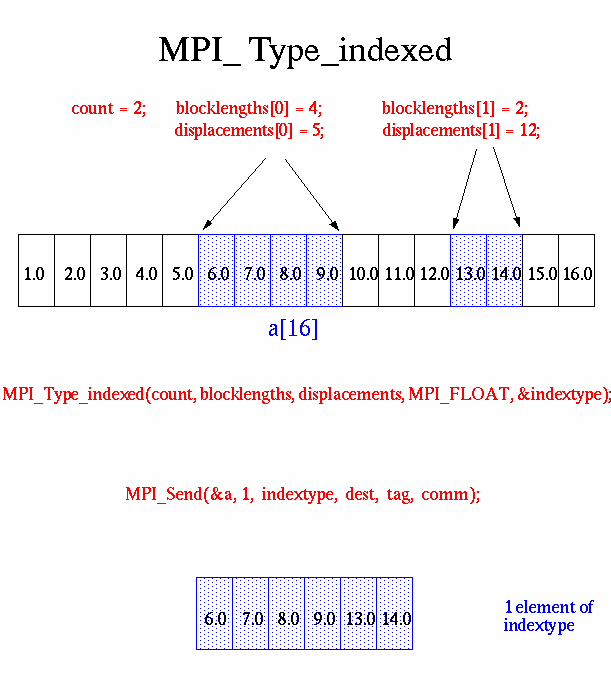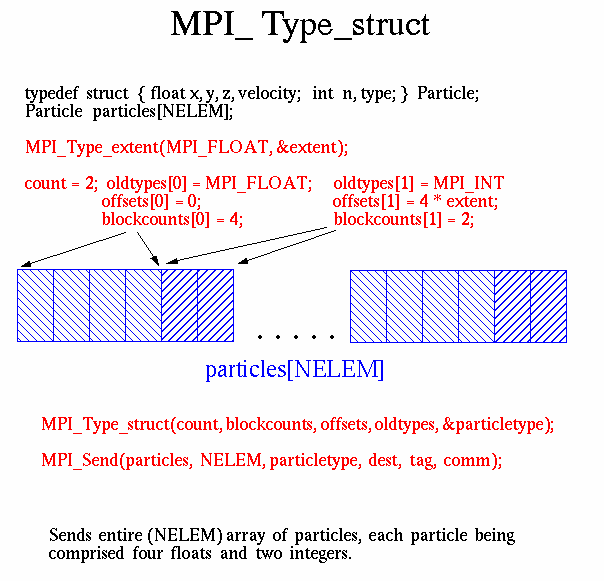# Derived Data Types

As previously mentioned, MPI predefines its primitive data types:

C Data TypesFortran Data Types
MPI_CHAR
MPI_WCHAR
MPI_SHORT
MPI_INT
MPI_LONG
MPI_LONG_LONG_INT
MPI_LONG_LONG
MPI_SIGNED_CHAR
MPI_UNSIGNED_CHAR
MPI_UNSIGNED_SHORT
MPI_UNSIGNED_LONG
MPI_UNSIGNED
MPI_FLOAT
MPI_DOUBLE
MPI_LONG_DOUBLE
MPI_C_COMPLEX
MPI_C_FLOAT_COMPLEX
MPI_C_DOUBLE_COMPLEX
MPI_C_LONG_DOUBLE_COMPLEX
MPI_C_BOOL
MPI_LOGICAL
MPI_C_LONG_DOUBLE_COMPLEX
MPI_INT8_T
MPI_INT16_T
MPI_INT32_T
MPI_INT64_T
MPI_UINT8_T
MPI_UINT16_T
MPI_UINT32_T
MPI_UINT64_T
MPI_BYTE
MPI_PACKED
MPI_CHARACTER
MPI_INTEGER
MPI_INTEGER1
MPI_INTEGER2
MPI_INTEGER4
MPI_REAL
MPI_REAL2
MPI_REAL4
MPI_REAL8
MPI_DOUBLE_PRECISION
MPI_COMPLEX
MPI_DOUBLE_COMPLEX
MPI_LOGICAL
MPI_BYTE MPI_PACKED

MPI also provides facilities for you to define your own data structures based upon sequences of the MPI primitive data types. Such user defined structures are called derived data types.

Primitive data types are contiguous. Derived data types allow you to specify non-contiguous data in a convenient manner and to treat it as though it was contiguous.

MPI provides several methods for constructing derived data types:

• Contiguous
• Vector
• Indexed
• Struct

### Derived Data Type Routines

MPI_Type_contiguous

The simplest constructor. Produces a new data type by making count copies of an existing data type.

``````MPI_Type_contiguous (count,oldtype,&newtype)
MPI_TYPE_CONTIGUOUS (count,oldtype,newtype,ierr)
``````

MPI_Type_vector

MPI_Type_hvector

Similar to contiguous, but allows for regular gaps (stride) in the displacements. MPI_Type_hvector is identical to MPI_Type_vector except that stride is specified in bytes.

``````MPI_Type_vector (count,blocklength,stride,oldtype,&newtype)
MPI_TYPE_VECTOR (count,blocklength,stride,oldtype,newtype,ierr)
``````

MPI_Type_indexed

MPI_Type_hindexed

An array of displacements of the input data type is provided as the map for the new data type. MPI_Type_hindexed is identical to MPI_Type_indexed except that offsets are specified in bytes.

``````MPI_Type_indexed (count,blocklens[],offsets[],old_type,&newtype)
MPI_TYPE_INDEXED (count,blocklens(),offsets(),old_type,newtype,ierr)
``````

MPI_Type_struct

The new data type is formed according to completely defined map of the component data types.

NOTE: This function is deprecated in MPI-2.0 and replaced by MPI_Type_create_struct in MPI-3.0

``````MPI_Type_struct (count,blocklens[],offsets[],old_types,&newtype)
MPI_TYPE_STRUCT (count,blocklens(),offsets(),old_types,newtype,ierr)
``````

MPI_Type_extent

Returns the size in bytes of the specified data type. Useful for the MPI subroutines that require specification of offsets in bytes.

NOTE: This function is deprecated in MPI-2.0 and replaced by MPI_Type_get_extent in MPI-3.0

``````MPI_Type_extent (datatype,&extent)
MPI_TYPE_EXTENT (datatype,extent,ierr)
``````

MPI_Type_commit

Commits new datatype to the system. Required for all user constructed (derived) datatypes.

``````MPI_Type_commit (&datatype)
MPI_TYPE_COMMIT (datatype,ierr)
``````

MPI_Type_free

Deallocates the specified datatype object. Use of this routine is especially important to prevent memory exhaustion if many datatype objects are created, as in a loop.

``````MPI_Type_free (&datatype)
MPI_TYPE_FREE (datatype,ierr)
``````

### Examples

#### Examples: Contiguous Derived Data Type

Create a data type representing a row of an array and distribute a different row to all processes.C and Fortran code examples here.

#### Examples: Vector Derived Data Type

Create a data type representing a column of an array and distribute different columns to all processes.C and Fortran code examples here.

#### Examples: Indexed Derived Data Type

Create a datatype by extracting variable portions of an array and distribute to all tasks.C and Fortran code examples here.

#### Examples: Struct Derived Data Type

Create a data type that represents a particle and distribute an array of such particles to all processes.C and Fortran code examples here.